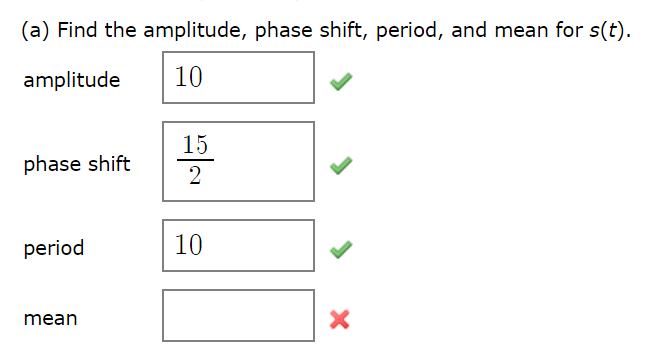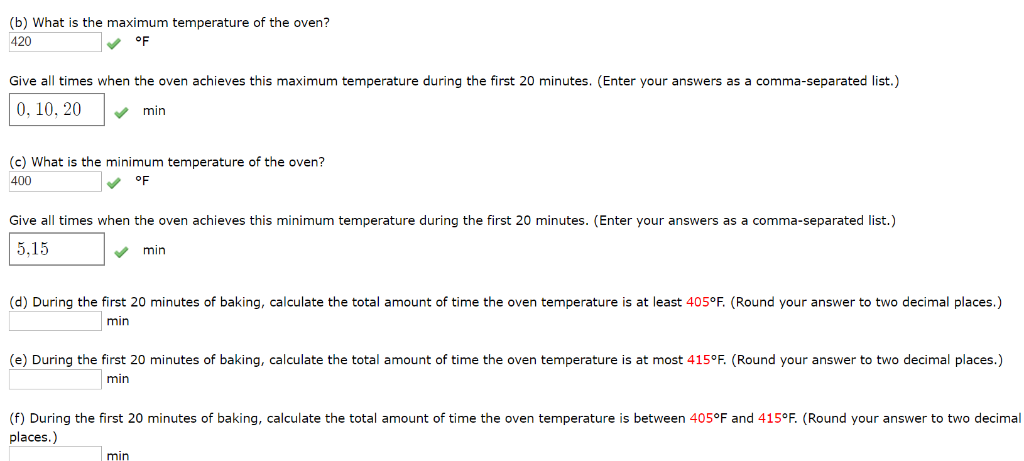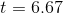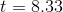# Hugo bakes world famous scones. The key to his success is a special oven whose temperature varies according to a sinusoi...

Hugo bakes world famous scones. The key to his success is a special oven whose temperature varies according to a sinusoidal function; assume the temperature (in degrees Fahrenheit) of the oven t minutes after inserting the scones is given by: y = s(t) = 10 sin (π/5t − 3π/2) + 410.

*** I need help finding the mean and parts D-F, please and thank you!(a) Find the amplitude, phase shift, period, and mean for s(t). 10 amplitude 15 phase shift 2 10 period mean
(b) What is the maximum temperature of the oven? 420 oF Give all times when the oven achieves this maximum temperature during the first 20 minutes. (Enter your answers as a comma-separated list.) 0, 10, 20 min (c) What is the minimum temperature of the oven? OF 400 Give all times when the oven achieves this minimum temperature during the first 20 minutes. (Enter your answers as a comma-separated list.) 5,15 min (d) During the first 20 minutes of baking, calculate the total amount of time the oven temperature is at least 405°F. (Round your answer to two decimal places.) min (e) During the first 20 minutes of baking, calculate the total amount of time the oven temperature is at most 415°F. (Round your answer to two decimal places.) min (f) During the first 20 minutes of baking, calculate the total amount of time the oven temperature is between 405°F and 415°F. (Round your answer to two decimal places.) min

we are givenCalculation of mean:now, we can find average of these two values(d)

we are given

S(t)=405

now, we can set it to and then we can solve for t.............Answer

(e)

we are given

S(t)=415

now, we can set it to and then we can solve for t.............Answer

(f)

we can find time between..............Answer

##### Add Answer of: Hugo bakes world famous scones. The key to his success is a special oven whose temperature varies according to a sinusoi...
More Homework Help Questions Additional questions in this topic.